## What value of x makes the equation 3x+7=22 true?

Question

What value of x makes the equation 3x+7=22 true?

in progress 0
8 months 2021-07-19T00:39:34+00:00 2 Answers 0 views 0

## Answers ( )

x = 5

Step-by-step explanation:

Subtract 7 from both sides:  3x + 7- 7 = 22 – 7

Simplify: 3x = 15

Divide both sides by 3

Simplify: x = 5

Hope this helps:)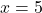Step-by-step explanation:

Given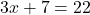, our goal is to isolate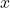such that will have an equation that tell usis equal to something.

Start by subtracting 7 from both sides: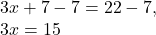Divide both sides by 3: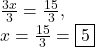Therefore, the value ofmakes the equationtrue.